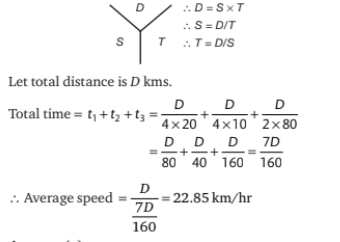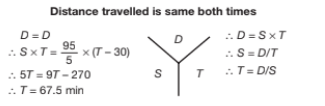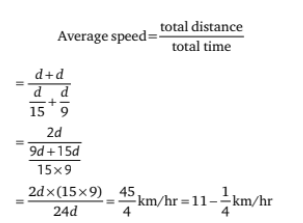# Mathematical Aptitude - Time and Distance

>>>>>>>>Time and Distance

• A

22.85 km• B

25.15 km• C

50 km• D

40 km• Option : A
• Explanation :• A

70.50 min• B

54 min• C

66.67 min• D

67.50 min• Option : D
• Explanation :• A

18 km• B

35 km• C

20 km• D

27 km• Option : A
• Explanation : Let distance be D
With speed 30km/hr he is 20 minutes late
With speed 45 km/hr he is 8 minutes late
∴ Difference between two time
= 20 - 8 = 12min = 12/60 hours
T = D/S
∴ D/30 - D/45 = 12/60
∴ D = 18km

• A

18 km• B

21 km• C

30 km• D

15 km• Option : A
• Explanation : Distance is same
∴ S1 × T1 = S2 × T2
∴ 24 X 6/60 = S2 X 8/60
To convert minutes to hours, divide minutes by 60
∴ S2 = 18 km/hr

• A

11 1/4• B

12 3/4• C

10 1/2• D

9 1/2• Option : A
• Explanation : Speed of the man in still water = 12 km/hr
Speed of the stream = 3 km/hr
Speed downstream = (12 + 3) = 15 km/hr
Speed upstream = (12 – 3) = 9 km/hr
Let the distance travelled be ‘d’ km Then## Description

Who can benefit –

• Any undergraduate or postgraduate student who is seeking Time and Distance objective type questions answers can use this section.
• Time and Distance Questions Answers can be used for the preparation of UGC NET Paper1 Exams.
• Time and Distance Questions are also used by students in the preparation of their Exams.
• All the teachers who are appearing for Kendriya Vidyalya Sangathan Entrance Exam PGT, TGT, can also use Time and Distance Multiple Choice Questions Answers.
• Time and Distance Questions – Mathematical Aptitudes can also be used by the students who wants to pursue pursuing B.Ed or M. Ed.
• Any student who wants to prepare for UGC NET Paper1 can also use this Time and Distance Questions .
• UGC NET first paper students can also prepare for their exams using Time and Distance Objective Type Questions Answers.
• You can get free access to Time and Distance Questions – Mathematical Aptitude pdf.
• Any student who wants to prepare for UGC NET Paper1, MBA Entrance Exam, CAT, MAT, SAT, Ph. D Entrance Exam, PET, NDA, CDS or any other competitive Exam can also use this Time and Distance Questions .

Various Search Terms used for this section are

• ## Time and Distance Questions for UGC NET Paper1

• ### Time and Distance Multiple Choice Questions For Competitive Exams

Related Quiz.
Time and Distance